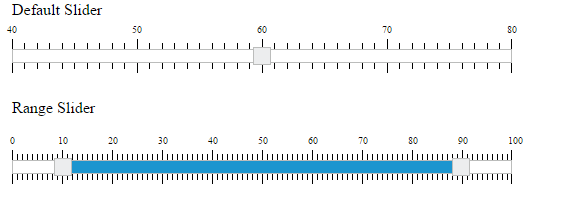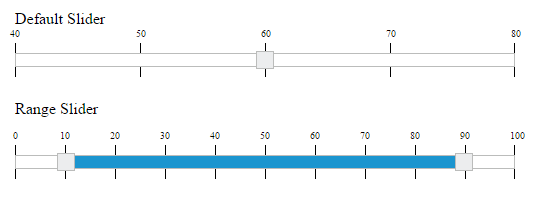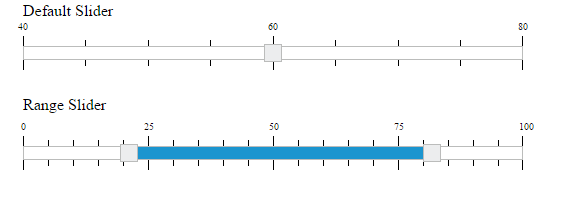# Scale Settings

14 Aug 20186 minutes to read

Slider widget includes option to display the scale on the Slider. Scale in Slider supports you to easily identify the current value/values of the Slider. Scale contains “small ticks” and “large ticks”. Values of the Slider is displayed above each large ticks.

## Show Scale

This property enables the scale in the Slider. By default, its value is “false”. Data type of this property is “Boolean”.

The following steps explains you the configuration of showScale property.

In an HTML page, specify the <div> elements to render the “Default Slider” and “Range Slider”.

• HTML
• ``````<div>Default Slider</div>
<ej-slider id='defaultSlider' [type]='default' [value]='value' [max]='maxValue'
[min]='minValue' [ticks]='ticks'></ej-slider>

<div>Range Slider</div>
<ej-slider id='rangeSlider' [type]='range' [value]='value' [ticks]='ticks'></ej-slider>``````
• HTML
• ``````<script>

import { Component } from '@angular/core';
import { SliderModule } from '@syncfusion/ej2-ng-inputs';

@Component({
selector: 'control-content',
templateUrl: 'app/components/slider/slider.component.html'',
})
export class DefaultSliderComponent {
public value: number = '60';
public range: string ='range';
public maxValue : string ='80';
public minValue : string ='40';
public ticks: Object = {
placement: 'both',
largeStep: 15,
smallStep: 5,
};
}
</script>``````

Execute the above code example to render the following output.## Enable Small Ticks

Slider widget provides you an option to enable/disable the small ticks present in the scale. By default, when you enable “showScale” property, small ticks is displayed in the scale. Use the showSmallTicks property to disable the small ticks present in the scale. Data type of this property is “Boolean”. The renderingTicks event will be triggered while creating each slider scale tick.

The following steps explains you on how to disable the small ticks in Slider.

In an HTML page, specify the <div> elements to render the “Default Slider” and “Range Slider”.

• HTML
• ``````<div>Default Slider</div>
<ej-slider id='defaultSlider' [type]='default' [value]='value' [max]='maxValue'
[min]='minValue' [ticks]='ticks'></ej-slider>

<div>Range Slider</div>
<ej-slider id='rangeSlider' [type]='range' [value]='value' [ticks]='ticks'></ej-slider>``````
• HTML
• ``````<script>

import { Component } from '@angular/core';
import { SliderModule } from '@syncfusion/ej2-ng-inputs';

@Component({
selector: 'control-content',
templateUrl: 'app/components/slider/slider.component.html'',
})
export class DefaultSliderComponent {
public value: number = '60';
public range: string ='range';
public maxValue : string ='80';
public minValue : string ='40';
public ticks: Object = {
placement: 'both',
largeStep: 15,
smallStep: 5,
showSmallTicks: true
};
}
</script>``````

Execute the above code example to render the following output.## Small step

This property specifies the distance between the two small ticks present in the scale and the position of the small ticks in the Slider scale. Data type of this property is “number”.

## Large step

This property specifies the distance between the two small ticks present in the scale and the position of the large ticks in the Slider scale. Data type of this property is “number”.

The following steps explains you on how to configure the smallStep and largeStep property in Slider scale.

In an HTML page, specify the <div> elements to render the “Default Slider” and “Range Slider”

• HTML
• ``````<div>Default Slider</div>
<ej-slider id='defaultSlider' [type]='default' [value]='value' [max]='maxValue'
[min]='minValue' [ticks]='ticks'></ej-slider>

<div>Range Slider</div>
<ej-slider id='rangeSlider' [type]='range' [value]='value' [ticks]='ticks'></ej-slider>``````
• HTML
• ``````<script>

import { Component } from '@angular/core';
import { SliderModule } from '@syncfusion/ej2-ng-inputs';

@Component({
selector: 'control-content',
templateUrl: 'app/components/slider/slider.component.html'',
})
export class DefaultSliderComponent {
public value: number = '60';
public range: string ='range';
public maxValue : string ='80';
public minValue : string ='40';
public ticks: Object = {
placement: 'both',
largeStep: 15,
smallStep: 5,
showSmallTicks: true
};
}
</script>``````

Execute the above code example to render the following output.In the above example, for “Default Slider” the “smallStep” value is specified as “5”, so for each 5 values from the starting value, small ticks is enabled. Also, “largeStep” value is specified as “20”, so for each 20 values from the starting value, large ticks is enabled.

Similarly for “Range Slider”, “smallStep” value is specifies as “5”, so for each 5 values from the starting value, small ticks is enabled. Also, “largeStep” value is specified as “25” so, for each 25 values large ticks is enabled.